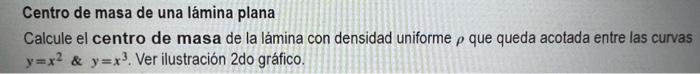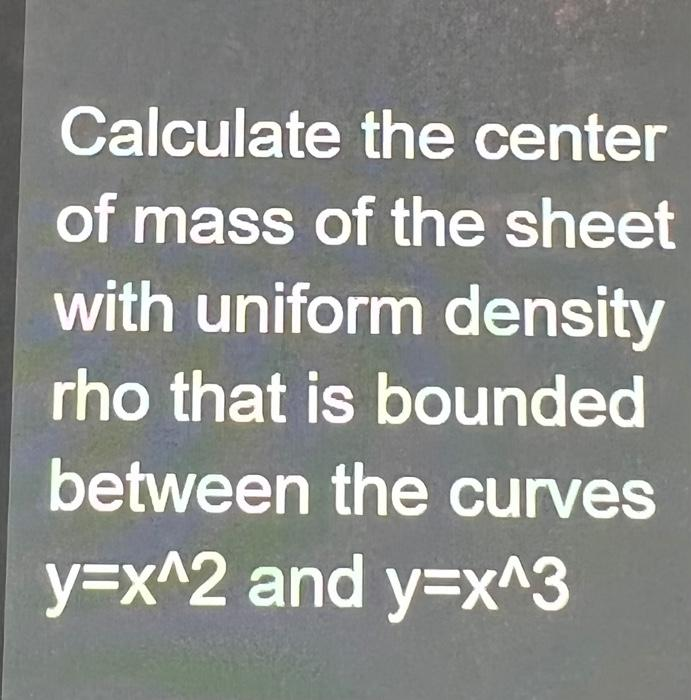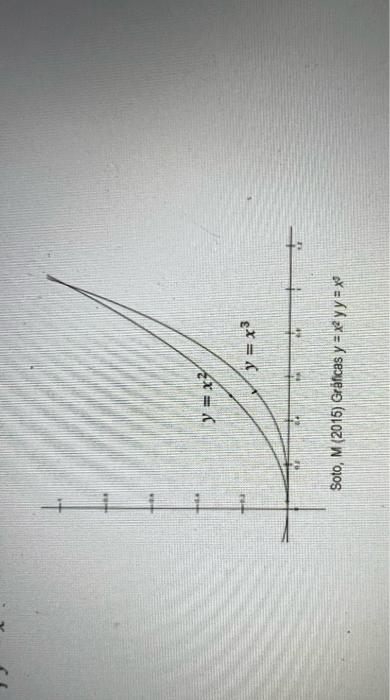# Question The original question is in spanish ive included the english translation. Use the image included to answer. Centro de masa de una lámina plana Calcule el centro de masa de la lámina con densidad uniforme p que queda acotada entre las curvas y=x² & y=x³. Ver ilustración 2do gráfico. Calculate the center of mass of the sheet with uniform density rho that is bounded between the curves y=x^2 and y=x^3 y = x² y = x³ + Soto, M (2015) Gráficas y = x² y y = x³